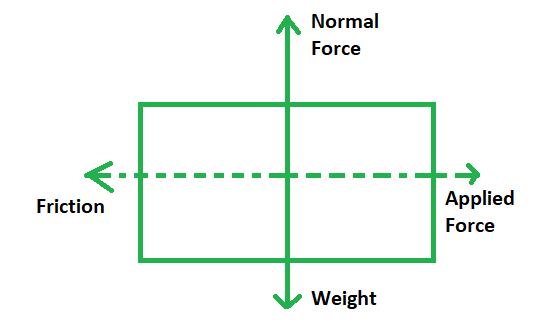# Friction Force Formula

• Last Updated : 08 Mar, 2022

Friction is the restraining force that operates in a direction opposite to that of the motion. Friction also generates heat. For example, if you shove a book across any plane surface, it will move. Furthermore, the power of the push propels the book, and as it slides across the surface, it slows down and comes to a halt. Furthermore, friction is the force that resists an object’s motion.

### Frictional ForceFrictional force is the force created when two surfaces come into touch with each other and slide against each other. These forces are mostly influenced by the surface roughness and the amount of force required when they are combined. The volume of frictional force is affected by the angle and location of the item. The forces of attraction are the primary cause of friction between objects. Friction is caused by shearing these “fused” joints and by the irregularity of the rougher surface tilling over the softer surface.

Formula

The following formula may be used to simply compute the highest level of frictional forces that ground can impart to an object:

Ffrict = µ x Fnorm

where,

• µ is the friction coefficient
• Fnorm refers to the normal force acting upon the said object, given as F = mg

### Sample Problems

Question 1. Assume that the mass of a car being pulled by a truck is 250 kg and the friction coefficient is 0.05. Find the magnitude of the frictional force acting upon the car.

Solution:

Given: m = 250 kg, µ = 0.05

Here, Fnorm = mg = 250 kg x 9.8 ms-1

= 2450 N

Now, Ffrict = µ x Fnorm

= 0.05 x 2450

Ffrict = 122.5 N

Question 2. Find the magnitude of frictional force acting upon a dead zebra being pulled into the water by a crocodile, if the mass of the said carcass is 80 kg and the coefficient of friction is 0.4.

Solution:

Given: m = 80 kg, µ = 0.4

Here, Fnorm = mg = 80 kg x 9.8 ms-1

= 784 N

Now, Ffrict = µ x Fnorm

= 0.4 x 784

Ffrict = 313.6 N

Question 3. Find the magnitude of frictional force acting upon a boat being pulled out of the water if its mass is 40 kg and the coefficient of friction is 0.7.

Solution:

Given: m = 40 kg, µ = 0.7

Here, Fnorm = mg = 40 kg x 9.8 ms-1

= 392 N

Now, Ffrict = µ x Fnorm

= 0.7 x 392

Ffrict = 274.4 N

Question 4. Which of these is higher: sliding friction or static friction?

Solution:

Since a sliding object has less time to interlock with the contact points on the floor, sliding friction is smaller than static friction. As a result, it is considerably easier to move an item that is already in motion than it is to start it.

Question 5. What is the relation between the weight of an object and the frictional force acting on it?

Solution:

The amplitude of the frictional force acting on an item is directly proportional to the weight of the body and is additionally affected by the plane’s inclination with respect to the horizontal. As a result, friction force is exactly proportional to body weight.

My Personal Notes arrow_drop_up